# BITSAT Mathematics Determinants MCQs

Refer to BITSAT Mathematics Determinants MCQs provided below. BITSAT Full Syllabus Mathematics MCQs with answers available in Pdf for free download. The MCQ Questions for Full Syllabus Mathematics with answers have been prepared as per the latest syllabus, BITSAT books and examination pattern suggested in Full Syllabus by BITSAT, NCERT and KVS. Multiple Choice Questions for Determinants are an important part of exams for Full Syllabus Mathematics and if practiced properly can help you to get higher marks. Refer to more Chapter-wise MCQs for BITSAT Full Syllabus Mathematics and also download more latest study material for all subjects

## MCQ for Full Syllabus Mathematics Determinants

Full Syllabus Mathematics students should refer to the following multiple-choice questions with answers for Determinants in Full Syllabus. These MCQ questions with answers for Full Syllabus Mathematics will come in exams and help you to score good marks

### Determinants MCQ Questions Full Syllabus Mathematics with Answers

#### Question: If a > 0, b > 0, c > 0 are respectively the pth, qth, rth terms of G.P., then the value of the determinant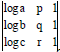is

• a) 0
• b) 1
• c) -1
• d) None of these

• a) 72
• b) 144
• c) 288
• d) 216

#### Question: If M (α) =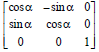M (β) =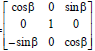then [M(α) M (β)]–1 is equal to -

• a) M(β) M (α)
• b) M (–α) M(–β)
• c) M(–β) M(–α)
• d) –M(β) M(α)

• a) 0
• b) 1
• c) 2
• d) 3

• a) 2ac
• b) – ac
• c) ac
• d) – 2ac

#### Question: The system of equations α x + y + z = α – 1, x + α y + z = α – 1, x + y + α z = α – 1 has no solution if α=

• a) –2
• b) α ≠ – 2
• c) Either – 2 or 1
• d) α = 1

• a) ω2
• b) 0
• c) 1
• d) ω

#### Question: If A =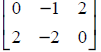, B =and M = AB, then find M–1.

• a)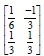• b)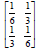• c)• d)#### Question: Inverse matrix of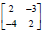• a)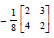• b)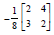• c)• d)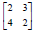#### Question: The equations 2x + 3y + 4 = 0; 3x + 4y + 6 = 0 and 4x + 5y + 8 = 0 are

• a) Consistent with unique solution
• b) Inconsistent
• c) Consistent with infinitely many solutions
• d) None of the above

• a) –2
• b) 4
• c) 2
• d) –4

#### Question: If a system of equation – ax + y + z = 0 x – by + z = 0; x + y – cz = 0 (a, b, c ≠ –1) has a non-zero solution then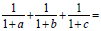• a) 0
• b) 1
• c) 2
• d) 3

• a) 0
• b) 1
• c) 2
• d) 4pqr

#### Question: Let α1, α2 and β1, β2 be the roots of ax2 + bx + c= 0 and px2 + qx + r = 0 respectively. If the system of equations α1y + α2z = 0 and β1y + β2z = 0 has a non-trivial solution, then

• a)• b)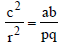• c)• d) None of these#### Question: If [ ] denotes the greatest integer less than or equal to the real number under consideration and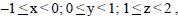then the value of the determinant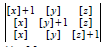is

• a) [z]
• b) [y]
• c) [x]
• d) None of these

• a) 1
• b)  α
• c) α2
• d) α3

#### Question: If the lines p1x + q1y = 1, p2x + q2y = 1 and p3x + q3y = 1 be concurrent, then the points (p1, q1), (p2, q2) and (p3, q3)

• a) Are collinear
• b) Form an equilateral triangle
• c) Form a scalene triangle
• d) Form a right angled triangle

#### Question: One of the roots of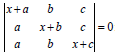is

• a) abc
• b) a + b + c
• c) –(a + b + c)
• d) –abc

Answer: –(a + b + c)

#### Question: The value of λ , for which the lines 3x - 4y =13, 8x -11y = 33 and 2x - 3y + λ = 0 are concurrent is

• a) –1
• b) –7
• c)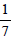• d) 9

#### Question: The determinant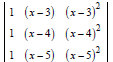vanishes for

• a) 3 values of x
• b) 2 values of x
• c) 1 values of x
• d) No value of x

#### Question: If the lines lx + my + n = 0, mx +ny + l = 0 and nx + ly + m = 0 are concurrent then

• a) l + m + n = 0
• b) l – m – n = 0
• c) l + m – n = 0
• d) m + n – l = 0

Answer: l + m + n = 0

#### Question: The equations 2x + 3y + 4 = 0; 3x + 4y + 6 = 0 and 4x + 5y + 8 = 0 are

• a) Consistent with unique solution
• b) Inconsistent
• c) Consistent with infinitely many solutions
• d) None of the above

#### Question: The value of the determinant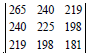is

• a) 1000
• b) 779
• c) 679
• d) 0

#### Question: If A =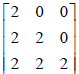then adj ( adj A) is equal to -

• a)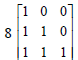• b)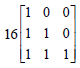• c)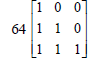• d) None of these• a) 213
• b) –231
• c) 231
• d) 39

## More Study Material

### BITSAT Full Syllabus Mathematics Determinants MCQs

We hope students liked the above MCQs for Determinants designed as per the latest syllabus for Full Syllabus Mathematics released by BITSAT. Students of Full Syllabus should download the Multiple Choice Questions and Answers in Pdf format and practice the questions and solutions given in above Full Syllabus Mathematics MCQs Questions on daily basis. All latest MCQs with answers have been developed for Mathematics by referring to the most important and regularly asked topics which the students should learn and practice to get better score in school tests and examinations. Studiestoday is the best portal for Full Syllabus students to get all latest study material free of cost.

### MCQs for Mathematics BITSAT Full Syllabus Determinants

Expert teachers of studiestoday have referred to NCERT book for Full Syllabus Mathematics to develop the Mathematics Full Syllabus MCQs. If you download MCQs with answers for the above chapter daily, you will get higher and better marks in Full Syllabus test and exams in the current year as you will be able to have stronger understanding of all concepts. Daily Multiple Choice Questions practice of Mathematics and its study material will help students to have stronger understanding of all concepts and also make them expert on all critical topics. You can easily download and save all MCQs for Full Syllabus Mathematics also from www.studiestoday.com without paying anything in Pdf format. After solving the questions given in the MCQs which have been developed as per latest course books also refer to the NCERT solutions for Full Syllabus Mathematics designed by our teachers

#### Determinants MCQs Mathematics BITSAT Full Syllabus

All MCQs given above for Full Syllabus Mathematics have been made as per the latest syllabus and books issued for the current academic year. The students of Full Syllabus can refer to the answers which have been also provided by our teachers for all MCQs of Mathematics so that you are able to solve the questions and then compare your answers with the solutions provided by us. We have also provided lot of MCQ questions for Full Syllabus Mathematics so that you can solve questions relating to all topics given in each chapter. All study material for Full Syllabus Mathematics students have been given on studiestoday.

#### Determinants BITSAT Full Syllabus MCQs Mathematics

Regular MCQs practice helps to gain more practice in solving questions to obtain a more comprehensive understanding of Determinants concepts. MCQs play an important role in developing understanding of Determinants in BITSAT Full Syllabus. Students can download and save or print all the MCQs, printable assignments, practice sheets of the above chapter in Full Syllabus Mathematics in Pdf format from studiestoday. You can print or read them online on your computer or mobile or any other device. After solving these you should also refer to Full Syllabus Mathematics MCQ Test for the same chapter

#### BITSAT MCQs Mathematics Full Syllabus Determinants

BITSAT Full Syllabus Mathematics best textbooks have been used for writing the problems given in the above MCQs. If you have tests coming up then you should revise all concepts relating to Determinants and then take out print of the above MCQs and attempt all problems. We have also provided a lot of other MCQs for Full Syllabus Mathematics which you can use to further make yourself better in Mathematics

Where can I download latest BITSAT MCQs for Full Syllabus Mathematics Determinants

You can download the BITSAT MCQs for Full Syllabus Mathematics Determinants for latest session from StudiesToday.com

Can I download the MCQs of Determinants Full Syllabus Mathematics in Pdf

Yes, you can click on the links above and download topic wise MCQs Questions PDFs for Determinants Full Syllabus for Mathematics

Are the Full Syllabus Mathematics Determinants MCQs available for the latest session

Yes, the MCQs issued by BITSAT for Full Syllabus Mathematics Determinants have been made available here for latest academic session

You can easily access the links above and download the Determinants Full Syllabus MCQs Mathematics for each topic

Is there any charge for the MCQs with answers for Full Syllabus Mathematics Determinants

There is no charge for the MCQs and their answers for Full Syllabus BITSAT Mathematics Determinants you can download everything free

How can I improve my MCQs in Full Syllabus Mathematics Determinants

Regular revision of MCQs given on studiestoday for Full Syllabus subject Mathematics Determinants can help you to score better marks in exams

What are MCQs for Full Syllabus Mathematics Determinants

Multiple Choice Questions (MCQs) for Determinants Full Syllabus Mathematics are objective-based questions which provide multiple answer options, and students are required to choose the correct answer from the given choices.